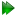The full CR72 2-site CPMG model

This is the model for 2-site exchange on most times scales (with the constraint that pA > pB), named after Carver and Richards (1972). It is selected by setting the model to CR72 full'. The equation is

 R2eff =R2A0 + R2B0 + kex -2νCPMGcosh-1D+cosh(η+) - D-cos(η-), (11.22)

where

 D± =±1 +, (11.23) η± = 2±Ψ +, (11.24) Ψ =R2A0 - R2B0 - pAkex + pBkex- Δω2 +4pApBkex2, (11.25) ζ = 2ΔωR2A0 - R2B0 - pAkex + pBkex. (11.26)

Note that these equations use the numerically simplified form derived in the appendix of Davis et al. (1994).

This model is not accurate when the motional process is very slow. In that case, the TSMFK01' model in Section 11.3.6 on pageshould be used instead.

The reference for this equation is: